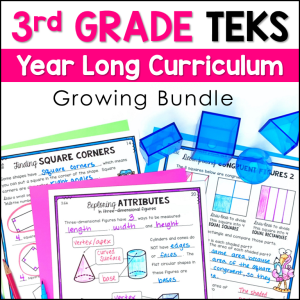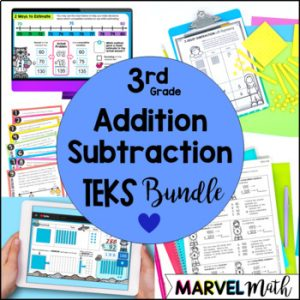Save 10% on your first order with code EXTRA10

# 3 Digit Subtraction with Regrouping #6 – Subtraction Circus

\$0.00\$134.25\$26.25\$12.00
SKU: 6922244 Category:

## Description

This 3-digit subtraction with regrouping resource includes 10 interactive Google Slides with engaging movable pieces and video instructions for students. This resource can be easily assigned in Google Classroom for math stations, independent practice or distance learning. This activity is perfect for 2nd grade and 3rd grade students.

You do not need Google Classroom to use this resource, but you do need a Google e-mail to use Google Slides. Homeschool parents can easily use this resource with their children by opening the Google Slides document.

Save 40% by purchasing the 3-Digit Subtraction with Regrouping Bundle!

You may also be interested in the 2-Digit Subtraction with Regrouping Bundle.

What’s Included?

• PDF with a Link to make a copy of the Google Slides in your Google Drive
• Video Instructions (Student devices must be able to access You-Tube)
• 2 self-checking math fact slides with all of the math facts students will use to solve the problems in the 2-digit subtraction practice section of this resource (24 math facts)
• 5 slides with 2-digit subtraction with regrouping practice (10 problems)
• 3 word problem slides (3 problems)
• Key

Topics include:

• Math Facts Warm-up
• Subtracting two 3-digit numbers that require regrouping in the tens place using the traditional algorithm
• Word Problems

Ideas on how to use this resource:

• Use it as a whole class teaching tool by projecting the slides and using the movable, interactive pieces to teach your lesson
• Use this resource as a no prep math station
• Use this resource for small group reteach or tutoring
• Assign this resource as a lesson for student practice as independent work
• Share the digital key for parents and students to check work at home